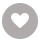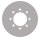水彩技法完全指南：贵图子的37堂实用水彩课电子书租阅

416人正在读 | 3人评论6.2

• 读书简介
• 目录
• 累计评论(4条)
• 读书简介
• 目录
• 累计评论(4条)

Chapter 1 水彩工具与用法

Chapter 2 跳跃的鲜果

Lesson 1 猕猴桃

Lesson 2 梨子

Lesson 3 桑葚

Lesson 4 火龙果

Lesson 5 红毛丹

Lesson 6 山竹

Lesson 7 草莓

Lesson 8 橙子与草莓

Lesson 9 苹果

Lesson 10 车厘子

Lesson 11 菠萝

Chapter 3 律动的蔬菜

Lesson 12 番茄

Lesson 13 冬笋

Lesson 14 百合根茎

Lesson 15 洋葱

Lesson 16 玉米

Lesson 17 胡萝卜

Lesson 18 卷心菜

Lesson 19 花椰菜

Lesson 20 蒜瓣

Lesson 21 蒜头

Lesson 22 生长

Lesson 23 白菜生长：第一阶段

Lesson 24 白菜生长：第二阶段

Lesson 25 白菜生长：第三阶段

Lesson 26 白菜生长：第四阶段

Chapter 4 花叶与花器

Lesson 27 玉吊钟

Lesson 28 蒂亚

Lesson 29 康乃馨

Lesson 30 桃叶鸦葱

Lesson 31 秘鲁百合

Lesson 32 鸢尾草

Lesson 33 猫耳草

Lesson 34 香槟玫瑰

Lesson 35 月季

Lesson 36 大岩桐

Lesson 37 西梅与青花瓷

01

￥36.15
02

WLOP
￥30.99
03

￥23.91
04

￥58.99
05

￥15.92
06

(日)中野京子
￥16.99
07

￥18.00
08

￥19.10
09

￥4.99
10

中国现代水墨画￥15.90

［英］史明理
￥15.90
•关注我们

最受欢迎的阅读产品

关注我们：

•关于我们

欢迎反馈宝贵意见给我们

客服书吧：当当云阅读问答